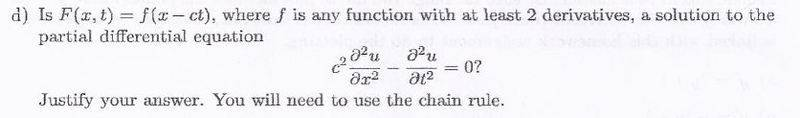# How to find verify solution to differential equation

digipony

## Homework Statement

See attached photo

## The Attempt at a Solution

I don't even know where to start on this problem. Usually, if I am given a possible solution to a DE, say y(t)=cos(t) as a solution to y''-y'+y=sin(t), I find whether it was a solution or not by manipulating it and then plugging it into the second equation that it may be a solution to and then seeing whether the resulting statement is true. For the ex. problem I give in this section, I would do this:
y'= -sint
y''= -cost
then sub them in: -cost +sint +cost = sint
and by simplifying get: sint = sint
so for this case, it y=cost is a solution.
But with the problem that is in the attached photo I have no idea what to do.

Does anyone have an guidance as to how I can solve the attached problem?
Thanks :)

#### Attachments

•diffeq.JPG
13.9 KB · Views: 481

Staff Emeritus
Homework Helper
Gold Member

## Homework Statement

See attached photo

## The Attempt at a Solution

I don't even know where to start on this problem. Usually, if I am given a possible solution to a DE, say y(t)=cos(t) as a solution to y''-y'+y=sin(t), I find whether it was a solution or not by manipulating it and then plugging it into the second equation that it may be a solution to and then seeing whether the resulting statement is true. For the ex. problem I give in this section, I would do this:
y'= -sint
y''= -cost
then sub them in: -cost +sint +cost = sint
and by simplifying get: sint = sint
so for this case, it y=cost is a solution.
But with the problem that is in the attached photo I have no idea what to do.

Does anyone have an guidance as to how I can solve the attached problem?
Thanks :)
Here's the "photo":Well, find $\displaystyle \frac{\partial}{\partial x} f(x-ct)$ and then the partial with respect to x of that.

Do the same with partials with respect to t.

Then plug all that in.

digipony
I don't have much experience with partial derivatives, am I on the right track here: the first partial derivative with respect to x, $$\displaystyle\frac{\partial}{\partial x} f(x-ct)$$ be: 1, therefore the second would be 0?

digipony
Sorry for the formatting on that, I had not even heard of LateX before finding this site.
Oh never mind this, it's fixed.

Last edited:
Staff Emeritus
Homework Helper
Gold Member
I don't have much experience with partial derivatives, am I on the right track here: the first partial derivative with respect to x, $$\displaystyle\frac{\partial}{\partial x} f(x-ct)$$ be: 1, therefore the second would be 0?

No.

$\displaystyle \frac{\partial}{\partial x} f(x-ct) = (1)\cdot f'(x-ct)\,,$ because d/dx (x) = 1 .

Similarly $\displaystyle \frac{\partial}{\partial t} f(x-ct) = (-c)\cdot f'(x-ct)\,.$

digipony
Oh, so that's what the reference to chain rule was about! Thank you!!